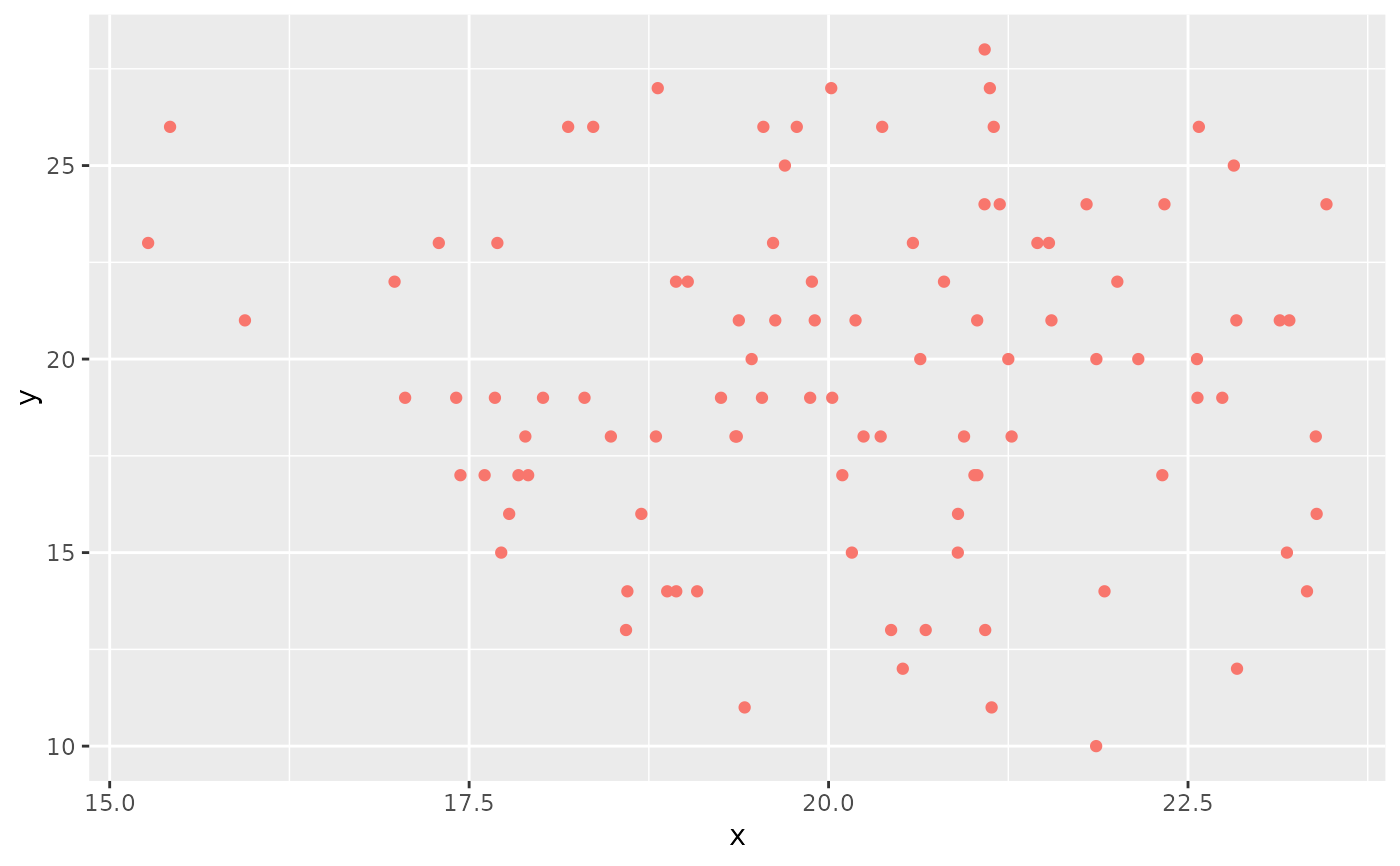Various themes to be applied to ggplot2-based plots

SeuratTheme

The curated Seurat theme, consists of ...

DarkTheme

A dark theme, axes and text turn to white, the background becomes black

NoAxes

Removes axis lines, text, and ticks

NoLegend

Removes the legend

FontSize

Sets axis and title font sizes

NoGrid

Removes grid lines

SeuratAxes

Set Seurat-style axes

SpatialTheme

A theme designed for spatial visualizations (eg PolyFeaturePlot, PolyDimPlot)

RestoreLegend

Restore a legend after removal

RotatedAxis

Rotate X axis text 45 degrees

BoldTitle

Enlarges and emphasizes the title

SeuratTheme()

CenterTitle(...)

DarkTheme(...)

FontSize(
x.text = NULL,
y.text = NULL,
x.title = NULL,
y.title = NULL,
main = NULL,
...
)

NoAxes(..., keep.text = FALSE, keep.ticks = FALSE)

NoLegend(...)

NoGrid(...)

SeuratAxes(...)

SpatialTheme(...)

RestoreLegend(..., position = "right")

RotatedAxis(...)

BoldTitle(...)

WhiteBackground(...)

## Arguments

...

Extra parameters to be passed to theme

x.text, y.text

X and Y axis text sizes

x.title, y.title

X and Y axis title sizes

main

Plot title size

keep.text

Keep axis text

keep.ticks

Keep axis ticks

position

A position to restore the legend to

## Value

A ggplot2 theme object

theme

## Examples

# Generate a plot with a dark theme
library(ggplot2)
df <- data.frame(x = rnorm(n = 100, mean = 20, sd = 2), y = rbinom(n = 100, size = 100, prob = 0.2))
p <- ggplot(data = df, mapping = aes(x = x, y = y)) + geom_point(mapping = aes(color = 'red'))
p + DarkTheme(legend.position = 'none')# Generate a plot with no axes
library(ggplot2)
df <- data.frame(x = rnorm(n = 100, mean = 20, sd = 2), y = rbinom(n = 100, size = 100, prob = 0.2))
p <- ggplot(data = df, mapping = aes(x = x, y = y)) + geom_point(mapping = aes(color = 'red'))
p + NoAxes()# Generate a plot with no legend
library(ggplot2)
df <- data.frame(x = rnorm(n = 100, mean = 20, sd = 2), y = rbinom(n = 100, size = 100, prob = 0.2))
p <- ggplot(data = df, mapping = aes(x = x, y = y)) + geom_point(mapping = aes(color = 'red'))
p + NoLegend()# Generate a plot with no grid lines
library(ggplot2)
df <- data.frame(x = rnorm(n = 100, mean = 20, sd = 2), y = rbinom(n = 100, size = 100, prob = 0.2))
p <- ggplot(data = df, mapping = aes(x = x, y = y)) + geom_point(mapping = aes(color = 'red'))
p + NoGrid()